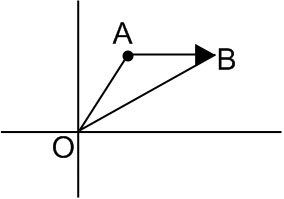Q

The value of , for which the points with position  vectors   respectively are the vertices of a right angled triangle at are

• Option 1)

2 and 1

• Option 2)

–2 and –1

• Option 3)

–2 and 1

• Option 4)

2 and –1

G gaurav

As we have learned

Scalar Product of two vectors -

- wherein

is the angle between the vectors

Position Vector -

If  and  are the position of vectors of two points A and B then

- wherein( a-2 )( a-1 )= 0

a = 1,2

Option 1)

2 and 1

Option 2)

–2 and –1

Option 3)

–2 and 1

Option 4)

2 and –1

Let be distinct non­-negative numbers. If the vectors   lie in a plane, then is

• Option 1)

the arithmetic mean of

• Option 2)

the geometric mean of

• Option 3)

the harmonic mean of

• Option 4)

equal to zero

G gaurav

As we have learned

Scalar Triple Product -

- wherein

Scalar Triple Product of three vectors .

Option 1)

the arithmetic mean of

Option 2)

the geometric mean of

Option 3)

the harmonic mean of

Option 4)

equal to zero

• Previous
• 1(current)
Exams
Articles
Questions